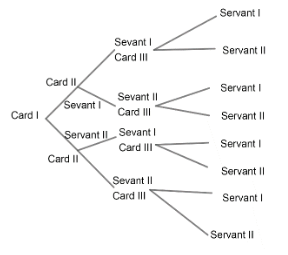Courses
Courses for Kids
Free study material
Free LIVE classes
MoreLIVE
Join Vedantu’s FREE Mastercalss

# A gentleman has 6 friends to invite. In how many ways can invitation cards be sent to them if he has three servants?Verified
361.5k+ views
Hint: For one person, invitation can be sent by 3 ways. So for $x$ persons, it can be sent in ${{3}^{x}}ways$.

Complete step by step solution:
Given, there are 3 servants.
So, one card can be sent by 3 servants, i.e. by 3 ways.
Let us understand this question by a smaller example where the number of cards is 3 and the number servants is 2. Let us draw a tree diagram for this situation.Therefore, the number of ways $=2\times 2\times 2={{2}^{3}}$.
Similarly, in the given question the number of cards to be sent out is 6 and the number of ways each card can be sent is 3.
Therefore, the total number of ways $=3\times 3\times 3\times 3\times 3\times 3$.
\begin{align} & ={{3}^{6}} \\ & =729 \\ \end{align}
Therefore, the number of ways in which six invites can be sent out if we have 3 servants to do the job is ${{3}^{6}}\ or\ 729$.

Note: In questions like these, the best way to solve them is to think of a similar example with smaller numbers and then use the same approach for the given question.

Last updated date: 28th Sep 2023
Total views: 361.5k
Views today: 3.61k